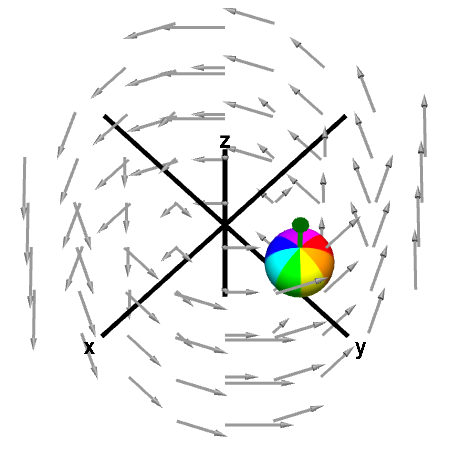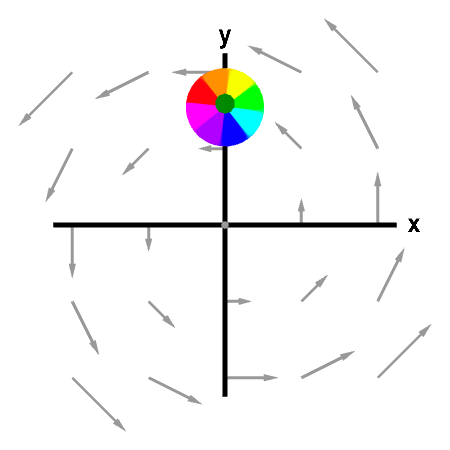# Math Insight

### Applet: A rotating sphere indicating the presence of curlThe rotation of the sphere when its center is fixed in an indication of the curl of the vector field. The curl of the vector field is shown by the green arrow. First panel shows the full vector field; second panel shows its projection in the $xy$-plane. You can move the sphere by dragging it with the mouse.

This vector field is $\dlvf(x,y,z)= (-y,x,0).$ Its curl is \begin{align*} \curl \dlvf(x,y,z) &= \left(\pdiff{}{y}0-\pdiff{}{z}x, \pdiff{}{z}(-y)- \pdiff{}{x}0, \pdiff{}{x}x - \pdiff{}{y}(-y) \right)\\ &=(0,0,2), \end{align*} which is a constant independent of point $(x,y,z)$ and always points in the positive $z$ direction. Therefore, the rotation of the sphere is unchanged as you move it around.